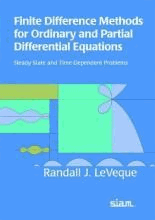# Finite Difference Methods for Ordinary and Partial Differential Equations : Steady-State and Time-dependent Problems by Leveque, Randall J.

• R 250.00
Unit price per
Tax included.

ISBN: 9780898716290

Society for Industrial & Applied Mathematics,U.S. | 06 Sep 2007

Paperback | 184 pages

This book introduces finite difference methods for both ordinary differential equations (ODEs) and partial differential equations (PDEs) and discusses the similarities and differences between algorithm design and stability analysis for different types of equations. A unified view of stability theory for ODEs and PDEs is presented, and the interplay between ODE and PDE analysis is stressed. The text emphasizes standard classical methods, but several newer approaches also are introduced and are described in the context of simple motivating examples. Exercises and student projects are available on the book's webpage, along with Matlab mfiles for implementing methods. Readers will gain an understanding of the essential ideas that underlie the development, analysis, and practical use of finite difference methods as well as the key concepts of stability theory, their relation to one another, and their practical implications. The author provides a foundation from which students can approach more advanced topics.